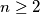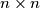### IMO Shortlist 2014 problem C3

Kvaliteta:
Avg: 0,0
Težina:
Avg: 7,5

Let$n \geq 2$ be an integer. Consider an$n \times n$ chessboard divided into$n^2$ unit squares. We call a configutaion of$n$ rooks on this board happy if every row and every column contains exactly one rook. Find the gretest positive integer$k$ such that for every happy configuration of rooks, we can find a$k \times k$ square without a rook on any of its$k^2$ unit squares.

(Croatia)

Izvor: https://www.imo-official.org/problems/IMO2014SL.pdf